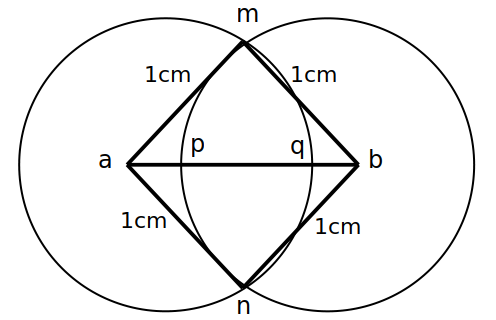Question 8

# Two identical circles intersect so that their centres, and the points at which they intersect, form a square of side 1 cm. The area in sq. cm of the portion that is common to the two circles is

SolutionWe know that quad ambn is a square of side 1.

Area of the sector a-mqn is $$\frac{90}{360}* \pi *1*1$$ = $$\frac{\pi }{4}$$.

Area of square = 1*1 = 1

Area of common portion = 2 * Area of sector - Area of square

= 2 * $$\frac{\pi }{4}$$ - 1 =  $$\frac{\pi }{2}$$ - 1